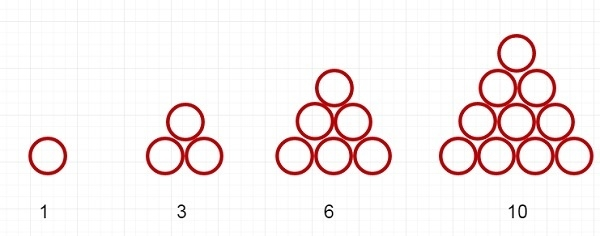# Check if a number can be represented as a sum of 2 triangular numbers in C++

In this section, we will see if we can express one number as the sum of two triangular numbers or not. The triangular numbers are like below −From the example, we can see that 1, 3, 6, 10 are some triangular numbers. We need to express a number N (say 16) as sum of two triangular numbers (6, 10).

The approach is very simple. We have to get all triangular numbers less than N. Form a set from these values. Now we have to take a number say X from the set, and check whether N – X is present in the set, then X can be represented as sum of two triangular numbers.

## Example

Live Demo

#include <iostream>
#include <set>
using namespace std;
bool isSumTriangularNum(int n) {
set<int> s;
int i = 1;
while (1) { //find and store all triangular numbers below n, and store into set
int x = i * (i + 1) / 2;
if (x >= n)
break;
s.insert(x);
i++;
}
for (auto x : s)
if (s.find(n - x) != s.end())
return true;
return false;
}
int main() {
int num = 16;
if(isSumTriangularNum(num)){
cout << "Can be represented";
}else{
cout << "Cannot be represented";
}
}

## Output

Can be represented